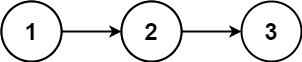# 382. Linked List Random Node

Medium
Given a singly linked list, return a random node's value from the linked list. Each node must have the same probability of being chosen.
Implement the `Solution` class:
• `Solution(ListNode head)` Initializes the object with the head of the singly-linked list `head`.
• `int getRandom()` Chooses a node randomly from the list and returns its value. All the nodes of the list should be equally likely to be chosen.
Example 1:Input
["Solution", "getRandom", "getRandom", "getRandom", "getRandom", "getRandom"]
[[[1, 2, 3]], [], [], [], [], []]
Output
[null, 1, 3, 2, 2, 3]
Explanation
Solution solution = new Solution([1, 2, 3]);
solution.getRandom(); // return 1
solution.getRandom(); // return 3
solution.getRandom(); // return 2
solution.getRandom(); // return 2
solution.getRandom(); // return 3
// getRandom() should return either 1, 2, or 3 randomly. Each element should have equal probability of returning.
Constraints:
• The number of nodes in the linked list will be in the range `[1, 104]`.
• `-104 <= Node.val <= 104`
• At most `104` calls will be made to `getRandom`.
• What if the linked list is extremely large and its length is unknown to you?
• Could you solve this efficiently without using extra space?

### 解題

/**
* type ListNode struct {
* Val int
* Next *ListNode
* }
*/
type Solution struct {
arr []int
}
arr := make([]int, 0)